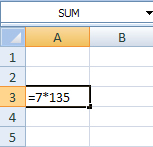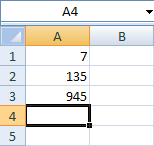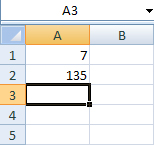# Formulas and Functions

Excel, like all spreadsheet programs, is designed to allow the user to perform calculations on large sets of data using built-in and user-defined functions.

## Operators

In all equations, operators are needed to define the desired action. Excel asserts the algebraic order of operations on all formulas. The basic operators needed for any equations include:

• Equality (=) is required of all formulas. Formula cells begin with equality, setting the cell equal to the given expression.
• Parentheses ( ) will contain an operation and calculate the value before the remaining expression.
• Exponential (^) will raise the first number to the power of the second.
• Multiplication (*) will calculate the product of two values or cells.
• Division (/) will calculate the fraction of two values or cells.
• Addition (+) will calculate the sum of two values or cells.
• Subtraction (-) will calculate the difference of two values or cells.

## Formulas

1. Select the active cell you wish to place your formula. The formula must begin with an equality sign.

2. Insert your expression and press enter.
Example:set A3 equal to the product of 7 and 135. Press enter to calculate the value.cell A3 has the contents =7*135cell A1 has the number 7, cell A2 has the number 135, and cell A3 has the number 945 (which is the product of 7*135)

## Cell Referencing

Spreadsheet programs, such as Excel, enable users to reference data from a cell or range of cells for use in calculations.

Each cell in the spreadsheet has a reference, given by its column and row position. In the Formulas section above, the eventual value, 945, is located in cell A3. A3 is the reference for the value 945 on that spreadsheet. For further calculations, instead of continuously using the original value, A3 can be substituted in its place.

Example:
Repeat the previous example using the values from above. Insert 7 in cell A1 and 135 in cell A2.cell A1 has the number 7 and cell A2 has the number 135

Insert “=A1*A2” to create a formula using cell referencing instead of inserting “=7*135” in A3.

It is important to understand that when referencing, the reference points to the cell and not the value inside. A1 tells Excel to look at A1 and pull whatever contents it holds. A1 does not tell the calculator to look for the value 7.

Example:
Change the value of A2 to 123.

The value of A3 has changed to 861, but the formula of the cell is still A1*A2.

## Functions

Excel is capable of performing a number of built in functions on a range of cells. To access these functions, use the Functions button.

To reference a range of cells, choose your starting cell and your ending cell.

A1:A4 – this continuous range includes cells A1, A2, A3, and A4.

A1:B3 – this continuous range includes cells A1, A2, A3, B1, B2, and B3

A1:A3, C1:C3 – this non-continuous range includes cells A1, A2, A3, C1, C2, and C3

• =sum(range) finds the sum of a range of values
• =average(range) finds the average of a range of values
• =min(range) finds the smallest value in a range
• =max(range) finds the largest value in a range
• =count(range) counts the number of cells in a range when the range contains numbers
• =counta(range) counts the number of cells in a range when the range contains any kind of data

## Autofill and Referencing

The autofill feature can be used to mass copy a formula. However, autofill works slightly differently when using referencing. When autofill is used with a reference, each cell in the autofill range will reference the cell adjacent to the previous reference.

Example:
Enter a value in the A1 cell. In the cell below it, enter the formula: =A1+1.

Use the autofill feature and drag the formula down a few cells. Select cell A3. Notice how its contains the formula: =A2+1

A3 will reference A2, and A4 will reference A3, and so on. Autofill will follow this formatting horizontally as well.

To perform a calculation on a constant value, users will need to signify the constant value. To do this in Excel, place a \$ between the column and row reference (=A\$1+1).

Autofill again, and select A3. Notice how A3 is now referencing A1.

Excel includes the capability to name or address a cell. For example, instead of having to constantly reference \$A\$10, the spreadsheet could reference a cell named monthly_income.

To address a cell, select the desired location and right-click the cell to select Define Name.

Define Names Pane

Within the pane, enter a name for your cell. You cannot use any name that contains a space, or is a reserved function name. Once you have entered a name, press OK.

Now you can reference your constant cell by its name. The autofill function will always reference the name of your cell if it is provided in the original formula.

## Removing a cell address

#### For Excel 2010

Click on the Formulas tab, then click on Manager. Then you can select the name and delete it.

#### For Excel 2013

Click on the Formulas tab, then click on Name Manager. Then you can select the name and delete it.